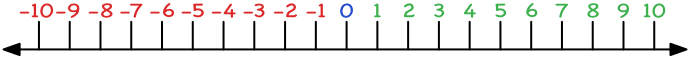Negative Numbers

# Negative Numbers

Basic,

Numbers greater than zero are normally shown without a sign. The number written as 5 has the same value as +5.

Negative numbers are numbers that are less than zero, and are shown with a minus sign. A number line, showing the position of numbers from -10 to +10, is shown below:The numbers on the left hand end of the scale are the smaller numbers. Working from left to right, numbers increase in value. -5 is larger than -8 because -5 is further to the right on the number line. Similarly, 2 is larger than -4.

## Examples

1. Which is the larger number, -6 or -2?